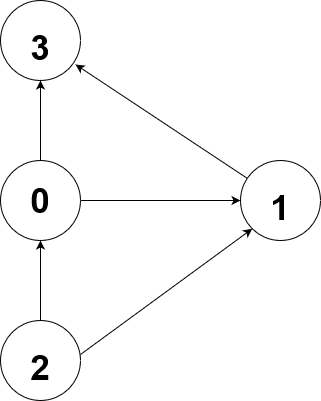Path Count in Directed Graph
Medium Accuracy: 25.32% Submissions: 3194 Points: 4

Given a directed graph and two vertices source and destination, your task is to complete the function countPaths(), whose function is to count the total number of ways or paths that exist between two vertices in a directed graph.
The Graph may be disconnected or may contain cycles, but the paths should not contain cycles.

Input Format:
The first line of the input contains an integer 'T' denoting the number of test cases. Then 'T' test cases follow. Each test case consists of three lines on input. The First line of each test case contains two integers 'N'
and 'M which denote the number of vertices and number of edges respectively. The Second line of each test case contains 'M space separated pairs u and v denoting that there is an edge from u to v. The Third line of each test case contains two space-separated integers source and destination, for which the count of the path is needed to be calculated.

Output Format:
For each testcase, in a new line, the count of all possible paths from source to destination will be printed.

This is a function problem so you only have to complete the given function countPaths that takes two integers source and destination as arguments. The method should return the count of all possible paths from source to destination.

Constraints:
1 <= T <= 100
1 <= N, M <= 100
0 <= u,v < = N-1

Example:
Input:

1
4 5
0 1 0 3 2 0 2 1 1 3
2 3

Output:
3
1

Explanation:
Testcase1:The total paths from 2 to 3 are:
2->1->3
2->0->3
2->0->1->3
So, a total of 3 paths.